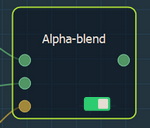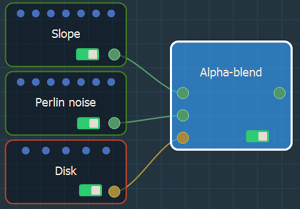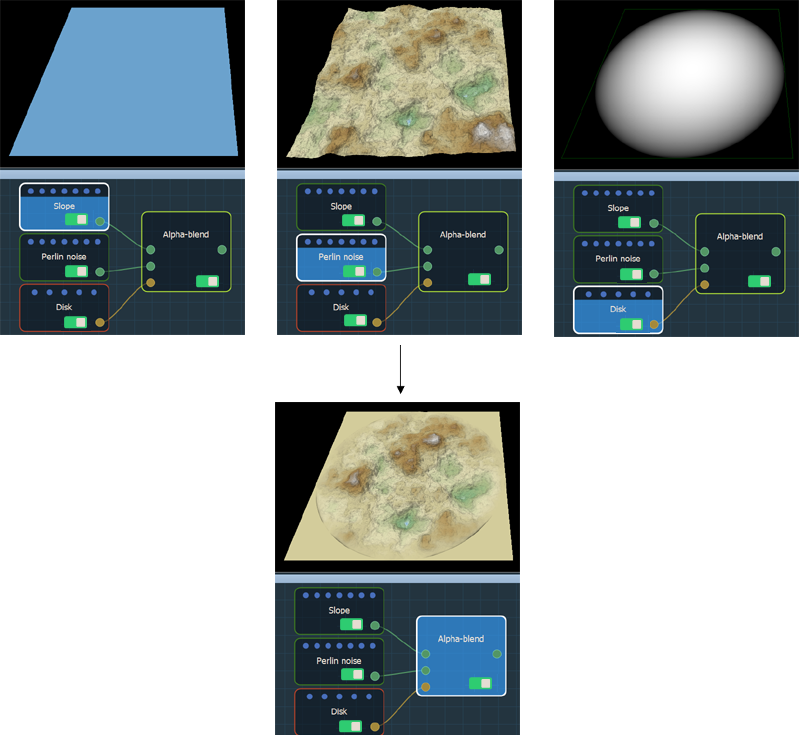This node creates an alpha composition between two terrains at locations defined by a mask.

The principle of the Alpha blend is as follows:

• In the black areas, the height of the first terrain is used.
• In the white areas, the height of the second terrain is used.
• Between the two, in the gray areas, the Alpha blend mixes both terrains.

When upgrading from Instant Terra v1.0 to v1.5, you need to modify your projects if they contain Alpha blend nodes to achieve the same result. If both entry terrains are the same size, simply swap them around. If they are not the same size, you need to invert the mask, which can be done by adding an Invert mask node.

To add a node, right click in the Graph Editor and select Create Node  > Terrain Composition > Alpha blend.Link the Alpha blend node to three input nodes: two terrain generators and a mask.At each vertex:

• If the value of the mask is equal to 0 (black areas), the height of the output terrain is equal to the height of the first input terrain. The second input terrain is therefore ignored for this vertex.
• If the value of the mask is equal to 1 (white areas), the height of the output terrain is equal to the height of the second input terrain. The first input terrain is therefore ignored for this vertex.
• If the value of the mask is between 0 and 1 (gray areas), the height of the first input terrain is multiplied by the subtraction of 1 and the value of the mask before being added to the height of the second input terrain multiplied by the value of the mask. The formula is mask (1 - mask) * terrain1 + mask * terrain2.

For example:Both input terrains and the input mask may have different sizes and resolutions. The size and resolution of the output terrain are determined by those of the first terrain, and the second terrain and the mask are enlarged or reduced so that their size corresponds exactly to the size of the output terrain.